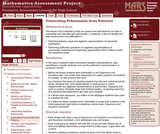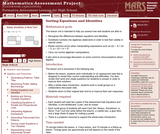# 2 Results

View
Selected filters:
• CCSS.Math.Content.HSA-APR.C.4Only Sharing Permitted
CC BY-NC-ND
Rating

This lesson unit is intended to help you assess how well students are able to manipulate and calculate with polynomials. In particular, it aims to identify and help students who have difficulties in: switching between visual and algebraic representations of polynomial expressions; and performing arithmetic operations on algebraic representations of polynomials, factorizing and expanding appropriately when it helps to make the operations easier.

نوع المادة:
التقييم
Lesson Plan
Provider:
Shell Center for Mathematical Education
U.C. Berkeley
Provider Set:
Mathematics Assessment Project (MAP)
04/26/2013Only Sharing Permitted
CC BY-NC-ND
Rating

This lesson unit is intended to help teachers assess how well students are able to: recognize the differences between equations and identities; substitute numbers into algebraic statements in order to test their validity in special cases; resist common errors when manipulating expressions such as 2(x Đ 3) = 2x Đ 3; (x + 3)_ = x_ + 3_; and carry out correct algebraic manipulations. It also aims to encourage discussion on some common misconceptions about algebra.

Material Type:
Assessment
Lesson Plan
Provider:
Shell Center for Mathematical Education
U.C. Berkeley
Provider Set:
Mathematics Assessment Project (MAP)